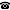2017 seminar talk: The exact strength of the class forcing theorem

Talk held by Kameryn Williams (Graduate Center, City University of New York (CUNY), USA) at the KGRC seminar on 2017-09-26.

Abstract

Gödel–Bernays set theory $\mathsf{GBC}$ proves that sufficiently nice (i.e. pretame) class forcings satisfy the forcing theorem—that is, these forcing notions $\mathbb P$ admit forcing relations $\Vdash_\mathbb{P}$ satisfying the recursive definition of the forcing relation. It follows that statements true in the corresponding forcing extensions are forced and forced statements are true. But there are class forcings for which having their forcing relation exceeds $\mathsf{GBC}$ in consistency strength. So $\mathsf{GBC}$ does not prove the forcing theorem for all class forcings. This is in contrast to the well-known case of set forcing, where $\mathsf{ZFC}$ proves the forcing theorem for all set forcings. On the other hand, stronger second-order set theories such as Kelley–Morse set theory $\mathsf{KM}$ prove the forcing theorem for all class forcings, providing an upper bound. What is the exact strength of the class forcing theorem?

I will show that, over $\mathsf{GBC}$, the forcing theorem for all class forcings is equivalent to $\mathsf{ETR}_\mathrm{Ord}$ the principle of elementary transfinite recursion for recursions of height $\mathrm{Ord}$. This is equivalent to the existence of $\mathrm{Ord}$-iterated truth predicates for first-order truth relative to any class parameter; which is in turn equivalent to the existence of truth predicates for the infinitary languages $\mathcal L_{\mathrm{Ord}, \omega}(\in, A)$ allowing any class parameter $A$. This situates the class forcing theorem precisely in the hierarchy of theories between $\mathsf{GBC}$ and $\mathsf{KM}$.

This is joint work with Victoria Gitman, Joel Hamkins, Peter Holy, and Philipp Schlicht.

Kurt Gödel Research Center for Mathematical Logic. Währinger Straße 25, 1090 Wien, Austria.+43-1-4277-50501. Last updated: 2010-12-16, 04:37.